﻿ Numerical Simulation of Flow around Diamond-Shaped Obstacles at Low to Moderate Reynolds Numbers

### Numerical Simulation of Flow around Diamond-Shaped Obstacles at Low to Moderate Reynolds Numbers

Seyed Reza Djeddi, Ali Masoudi, Parviz GhadimiOPEN ACCESSPEER-REVIEWED

## Numerical Simulation of Flow around Diamond-Shaped Obstacles at Low to Moderate Reynolds Numbers

Seyed Reza Djeddi1, Ali Masoudi1, Parviz Ghadimi1,1Department of Marine Technology, Amirkabir University of Technology, Tehran, Iran

### Abstract

In this paper, viscous fluid flow over an unconventional diamond-shaped obstacle in a confined channel is simulated in low to moderate Reynolds numbers. The diamond-shaped obstacle is altered geometrically in order to represent different blockage coefficients based on the channel height and different aspect ratios based on the length to height ratios of the obstacle. An in-house finite difference Navier-Stokes solver using staggered grid arrangement and Chorins projection method is developed for the simulation of the laminar viscous flow. The numerical solver is validated against numerical results that are presented in the literature for the flow over rectangular cylinders and good agreement is observed. Grid resolution has been studied within a mesh convergence test and as a result, suitable grid dimension is achieved. A series of simulations have been carried out for each set of geometry and configuration in order to find the critical Reynolds number for each case in which the vortex shedding will occur. Therefore, simulations are divided into two groups of steady and unsteady flows. In the case of unsteady flow, non-dimensional Strouhal Number (St) is investigated and results prove the dependency of St on the blockage coefficient and aspect ratio. It is shown that the Strouhal number will increase with the rise of blockage ratio and the local maximum of St will occur at lower Re for geometries with lower aspect ratios (bluff bodies) than geometries with higher aspect ratios, i.e. with more streamlined bodies.

### At a glance: Figures

123456
Prev Next

• Djeddi, Seyed Reza, Ali Masoudi, and Parviz Ghadimi. "Numerical Simulation of Flow around Diamond-Shaped Obstacles at Low to Moderate Reynolds Numbers." American Journal of Applied Mathematics and Statistics 1.1 (2013): 11-20.
• Djeddi, S. R. , Masoudi, A. , & Ghadimi, P. (2013). Numerical Simulation of Flow around Diamond-Shaped Obstacles at Low to Moderate Reynolds Numbers. American Journal of Applied Mathematics and Statistics, 1(1), 11-20.
• Djeddi, Seyed Reza, Ali Masoudi, and Parviz Ghadimi. "Numerical Simulation of Flow around Diamond-Shaped Obstacles at Low to Moderate Reynolds Numbers." American Journal of Applied Mathematics and Statistics 1, no. 1 (2013): 11-20.

 Import into BibTeX Import into EndNote Import into RefMan Import into RefWorks

### 1. Introduction

Flow around bluff and streamlined bodies has attracted many scientists and researchers for long period of time. The importance of this problem can be sought in its vast applications in engineering problems. Separation and vortex shedding are the common phenomena which happen at relatively low Reynolds numbers as a result of high positive pressure gradients. Vortex shedding causes fluctuation of pressure distribution on the body surface. Due to the vortex-induced forces, the body oscillates with a definite frequency. This may cause damages to structures such as suspended bridges and offshore oil platforms. Despite of many studies which have been done on flow around circular cylinders, rectangular shaped obstacles have been studied less experimentally and numerically, however, these shapes have many applications in analysis of aerodynamics of structures and fluid-structure interaction (FSI) problems. In the problem of flow around a confined cylinder in addition to the common parameters such as Reynolds number, another parameter called blockage coefficient (which is defined as the ratio of square side length to the channel height) should be considered .

Based on Reynolds number, different regimes of flow can be observed for the problem of flow around confined rectangular cylinder . At very low Reynolds numbers (Re << 1), viscous forces dominate the flow. In this creeping flow, no separation occurs. With the increase of Reynolds number, flow separates at trailing edge and forms a recirculation region which consists of two symmetric vortices. Size of recirculation zone increases with an increase of Reynolds number and by reaching the critical Reynolds number, Von Kármán vortex street with periodic vortex shedding happens. With further increase of Reynolds number beyond this critical value, separation will occur in the leading edge, but the range of Re for this phenomenon has not been studied clearly and only the range of 100-150 is reported [2, 3].

Okajima  has investigated the Strouhal number for aspect ratios (ratio of cylinder’s length to height) of 1 to 4 and a range of Reynolds numbers and stated the commence of periodic vortex motion at Reynolds number of 70 leading to an upper limit of critical Reynolds number at Re ≈ 70. A smaller value of critical Reynolds number Re ≈ 54 was determined by Klekar and Patankar  based on a stability analysis of the flow. Davis et al.  interpreted flow around a rectangular cylinder for a wide range of Reynolds numbers and aspect ratios of 1/4 and 1/6. Two-dimensional study of this problem for Reynolds numbers of 90 to 1200 and aspect ratios of 1/8 and 1/4 has been done by Mukhopadhyay et al. . Also, Suzuki et al.  has investigated this problem for a range of Reynolds numbers from 56.3 to 225 and aspect ratios of 1/20 to 1/5. Sohankar et al.  have studied the effects of blockage coefficient on an unsteady 2-D laminar flow at different angles of incident for Reynolds numbers of 100 and 200. Moreover, experimental and numerical analyses have been carried out by Nakamura et al.  for low Reynolds numbers. Experiments have been carried out at a low speed wind tunnel and the numerical analysis was based on the finite difference method of 2-D Navier-Stokes equations. The aspect ratio of the tested rectangular cylinders ranged from 3 to 16 in the experiments and 3 to 10 in the numerical computations.

Contrary to the flow around circular cylinder in which Reynolds number of 180 is introduced as the starting point for the formation of 3D structures in the wake, there has not been an accurate and comprehensive study on this phenomenon for flow around rectangular cylinders. As a hint, Reynolds number of 300 has been introduced by Franke  as starting point of this phenomenon. Breuer et al.  stated that this criterion is slightly beyond the limit of 2D simulation and the disturbances which occur in the periodic nature of 2D vortex shedding, has proved this theory. They studied flow around rectangular cylinder for the blockage coefficient of 1/8 and by two different methods of finite volume method (FVM) and lattice-boltzmann method (LBM). The experimental studies of Dutta et al  has verified the results of Breuer et al  and showed that beyond the Reynolds number of 200 aspect ratio of cylinder has to be taken into account due to the formation of 3D structures. One of the most recent studies in this field has been carried out by Berrone et al.  by means of adaptive finite element method (AFEM) and FVM for aspect ratios of 1 to 5 and Reynolds numbers of 100, 400 and 1000.

For higher Reynolds numbers, numerical modelings have been done by different methods like large eddy simulation (LES) and high expensive computational method of direct numerical simulation (DNS). As an example, Bruno et al.  studied flow around a cylinder at Re = 40,000 by means of a finite volume discretization method. John and Rang  applied finite element method to the large eddy simulations of the flow past a square cylinder at Re = 22,000. Other studies have been done by Xin liang et al.  and Kogaki et al. .

Dutta et al.  have experimentally studied flow past a square cylinder placed at an angle of incidence using particle image velocimetry, hot wire anemometry, and flow visualization. Data for four cylinder orientations θ=0, 22.5, 30, and 45° (diamond-shaped) and two aspect ratios of 16 and 28 have been reported. Reynolds numbers ranging 200 to 410 have been chosen. Results are presented in terms of drag coefficient, Strouhal number, time averaged velocity, stream traces, turbulence intensity, powerspectra, and vorticity field. A minimum of drag coefficient and maximum of Strouhal number is reported at a cylinder orientation of θ=22.5°. The effect of the aspect ratio has been studied and the results proved the formation of 3D structures and entrance to turbulent zone for Re > 200.

For the current problem of flow around diamond-shaped obstacle another investigation has been done by W. Regulski and Szumbarski . They applied Lattice-Boltzmann approach and studied flow around circular and diamond-shaped obstacle in the blockage coefficient of 0.4 with the use of a Spectral Element Method (SEM) in the regime of low and moderate Reynolds numbers ranging from 10 to 200. The velocity profiles for the channel are reported and Strouhal number of the Von Kármán vortex street behind the cylinder and the pressure drop is calculated for different Reynolds numbers.

In the present study, 2D laminar flow around a confined diamond-shaped obstacle has been investigated using a finite difference Navier-Stokes solver. Five different geometries have been studied in a range of different Reynolds numbers starting from fully viscous or creeping flow at Re = 1, subcritical Reynolds numbers, critical Reynolds number Recr and super critical Reynolds numbers up to the limit of 2D simulations, i.e. Re ≤ 200.

Mesh dependency study has been carried out for a set of four different grid resolutions which includes minimum cell sizes of Δh = 0.05, 0.01, 0.005, 0.001 mm.

Validation of results is carried out using the present data for the problem of flow around rectangular cylinder in a range of subcritical Re and blockage coefficient of 1/8 .

Governing equations and the numerical procedure are discussed in section 2. In section 3, the computational domain, geometry configurations, boundary conditions along with mesh dependency and validation tests are presented. Section 4 includes the numerical results from flow simulation in both steady and unsteady regimes. Investigations on the critical Reynolds number and the St in the regime of unsteady flow are presented in sections 4.2 and 4.2.1, respectively. A discussion and conclusion on the obtained results are offered in section 5.

### 2. Description of Numerical Method

Numerical representation of fluid flow dynamic is sought through a set of governing formula known as Navier-Stokes equations. The laminar viscous flow in 2-dimensional framework is written in form of two momentum equations and a continuity equation which satisfies the conservation of mass in the computational domain and within the fluid. The non-dimensional governing equations are the momentum equations as in(1)

and the continuity equation for the conservation of mass as in(2)

in which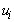are the velocities,is the pressure andis the external forces acting on the fluid. In the non-dimensional form of equations as stated above, Re is the Reynolds number that can be calculated using the fluid properties, velocity and a characteristic length as follows:(3)

Here,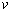is the dynamic viscosity of the fluid. In order to overcome the problem of oscillating pressure field, a staggered grid arrangement is used in which the pressure data is located at the cell centers and theand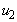velocities are placed on the vertical and horizontal cell faces, respectively. This type of variable positioning has an infamous alternative called co-located grids  for which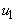,and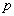are all evaluated at cell centers and a finite volume-based discretization employs special interpolation schemes to determine the flux across the cell edges. It is well-known that a naïve co-located grid arrangement leads to a decoupled (checkerboard) pressure field and oscillations in solutions. Therefore, in the present study, the staggered grid arrangement is chosen for the positioning of the primitive variables. An equidistant orthogonal grid with the help of a flagging technique is used in order to distinguish obstacle cells from fluid cells in which the governing equations should be solved.

Using a finite difference discretization scheme, the momentum equation can be discretized in both space and time. An Euler explicit (forward differencing) temporal discretization along with a chorin’s projection method, originally introduced by Chorin  is used to solve the time-dependant (transient) incompressible fluid-flow. Using this formulation, intermediate velocities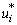are calculated explicitly using the momentum equation while ignoring the pressure gradient in the right-hand-side of the equation.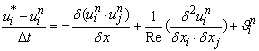(4)

in whichis the velocity at the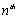time level and the intermediate velocities,, do not satisfy the continuity equation. By taking the divergence-free (continuity) condition, a Poisson’s equation for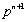is derived as in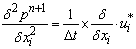(5)

This equation is solved using the following boundary condition for the pressure at thetime level.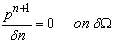(6)

in which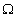is the domain boundary. After solving the Poisson’s equation, the projection step is performed in which the velocities at the next time level are calculated using the following equation.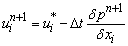(7)

One of the main advantages of the Chorin’s projection method is that the calculated velocity field is forced to satisfy the continuity equation in each time step.

In the present study, an in-house computer code is developed using the above mentioned methodology and algorithm. A schematic of the generated staggered grid and the location of the primitive variables are depicted in Figure 1.

Figure 1. Staggered grid and the location of primitive variables inside the computational domain

Fluid and Obstacle cells are defined in the initialization step and the obstacle cells that serve as boundaries are flagged based on their location with respect to the adjacent fluid cells.

In the present study, study of the flow around an obstacle in a confined channel, is aimed. Therefore, an inlet/outlet configuration has to be implemented on the upstream and downstream boundaries, respectively. The inflow boundary condition at the upstream uses an explicitly given inlet velocity while the outflow boundary condition at the other end of the channel is set in a way that the normal derivatives of both velocity components will vanish. The latter boundary condition means that the total velocity will not change in the direction normal to the outflow boundary and therefore can act as a non-reflective exit boundary. On the other hand, the no-slip wall boundary condition will force the continuous velocities to vanish in order to satisfy the no-slip condition in a way that all the tangential velocities for the locations lying directly on the boundary of a non-moving wall will have zero value.

The computer code uses a successive over-relaxation (SOR) iterative method to solve the Poisson’s equation for the next time level pressure values based on the intermediate velocities. The iterative loop will help the conservation of mass to be satisfied.

Although, the solution algorithm is easily implemented and the orthogonal equidistant grid leads to many advantages in storage and simplicity of computer code, the explicit time integration that was discussed above will impose some restrictions on time step size that has to be used for the numerical solution of the equations. This restriction is due to the stability reasons and based on the Courant-Friedrichs-Levi (CFL) criterion, in order to preserve a maximum courant number (CN) of 1.0, the following restriction of(8)

has to be fulfilled which is based on the courant number definition as follows: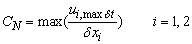(9)

in which,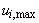denotes the maximum velocity indirection.

In the solution algorithm, first the initial and boundary conditions are set. Then, the intermediate velocities will be calculated using the time-explicit finite difference discretized momentum equations in the absence of pressure gradients. The intermediate velocities will then be used to solve the Poisson pressure equation by SOR method. Afterwards, the pressure gradients at the new time level will be used to calculate velocities at the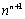time level through a projection step. The algorithm will continue until a desired final time is reached.

### 3. Details of the Test Case

3.1. Geometry of the Computational Domain

In this section, the computational domain and different geometries and configurations of the obstacle are presented. The diamond-shaped obstacle used for these studies has 5 different shapes based on the aspect ratio and the respective blockage ratio. In the standard form, hereafter referred to as ST, the obstacle has the shape of a rhombus or an equilateral quadrilateral with equal four sides. It can also be seen as a shifted rectangular cylinder which has been rotated 45 degrees. The diameter in ST shape is set in a way that satisfies the blockage ratio of 0.2. The obstacle is then stretched in both vertical and horizontal directions by multiplying each of the two diagonals by 1.5 and 2.0 in a way that 4 different geometries with different aspect ratios can be generated. Spanwise and streamwise stretched geometries are called H1, H2 and L1, L2, respectively. Different geometries and the computational domain are depicted in Figure 2.

It can be seen in Figure 2 that the diamond-shaped obstacle is placed in the middle of a confined channel with equal distances from both side-walls. An inlet boundary is placed at a distant 1.5H, upstream of the obstacle with H being the height of the channel. With the inradius (radius of the incircle) of the diamond obstacle being 0.2H, it can be inferred that the inlet boundary is far enough from the obstacle based on the respective hydraulic diameter (D) of the obstacle, with the distance being close to 8D. The outflow or exit boundary is also placed far away from the obstacle with the distance of 4.55H or almost 23D. Geometrical details of the 5 different obstacles are presented in Table 1. The aspect ratio is defined as the ratio of streamwise length to the spanwise length.

#### Table 1. Geometrical details of five different obstacles

3.2. Boundary Conditions

General boundary conditions for the solution of the Navier-Stokes equations have been presented in section 2. Stokes’s no-slip boundary condition is applied to the side-walls of the channel in which the tangential velocity on the nodes lying directly on the wall is set to zero or the relevant wall velocity (for the case of moving wall). Here, the fixed no-slip wall condition requires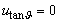on the wall boundary nodes.

For the inlet boundary, a parabolic velocity profile is prescribed at the entrance of the channel in order to simulate a fully developed laminar flow inside the confined channel and upstream of the diamond cylinder. For the sake of simplicity in the calculation of the flow’s Reynolds number, the maximum velocity of the parabolic profile is set to be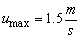in order to achieve the similar uniform velocity of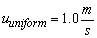.

The outlet boundary condition for the flow inside a channel with inflow/outflow boundaries at each end, needs careful attention in terms of numerical representation. However, due to the large computational domain that is set downstream of the diamond cylinder, the solution of the flow in vicinity of the obstacle will not be affected. Nevertheless, the zero gradient of the normal velocity at the exit boundary is forced inside the solution algorithm.

3.3. Validation and Mesh Dependency Study

The numerical procedure introduced and explained in the previous sections has to be validated in order to make certain about its accuracy and efficiency. For this purpose, flow around the rectangular cylinder is solved for a range of low Reynolds numbers for which a steady solution is possible. No experimental results is available in the literature for 2-dimensional low Reynolds number flow around a square cylinder. Therefore, viscous laminar flow over rectangular cylinder is compared against the numerical results of Breuer et al.  using Finite Volume Method (FVM). The blockage ratio for the case of the square cylinder is set to be B = 1/8. The critical Reynolds number for the rectangular cylinder in which the vortex shedding will occur is around 60 as stated in different studies presented in the literature. As a result, flow around the rectangular cylinder is simulated in a range of low Reynolds numbers from 5 to a little less than 60 (i.e. 58) with the step of 5. The recirculation length as the length of the closed near-wake is measured for different test cases and the results are shown in Figure 3. The results are in very good agreements with the results of Breuer et al.  using a FVM method. The non-dimensional recirculation lengths based on the square hydraulic diameter (D) at different Reynolds numbers are plotted and a curve fit on the results give a linear relationship between the recirculation length and the Reynolds number which is very close to the results of previous studies.

In order to achieve mesh independent results, a mesh dependency study is done for the current case. This mesh convergence test is based on the diamond-shaped obstacle placed in the middle of the flow inside the confined channel. Four different grids are generated from the coarsest grid which consists of 40,000 cells to the finest grid with 2,560,000 cells. Different grids that were used for the mesh dependency test are listed in Table 2. This test is performed with respect to the validation test which was described earlier. The respective errors for the recirculation length in comparison with the results of Breuer et al.  are mentioned in the last column of Table 2.

#### Table 2. Grids used for the mesh convergence test

Table 2 shows that, by further grid refinement (Grid 4), no major improvement is achieved in the numerical results and therefore, the fine grid (Grid 3) with the grid size of 0.005m (5mm) will be sufficient for the accurate and efficient simulation of the flow around the diamond-shaped obstacle. Another result was that by using the coarsest grid, the calculated recirculation length is smaller than that of the medium, fine and finest grids.

### 4. Results and Discussions

Flow around the diamond-shaped obstacle has been simulated at a Reynolds number range 1 ≤ Re < 200 using the in-house finite difference Navier-Stokes solver code, 2D_NAVISTO. The laminar viscous flow is solved for different geometrical representations of a diamond-shaped obstacle. The Reynolds number is adjusted by altering the fluid’s dynamic viscosity and keeping the obstacle’s hydraulic diameter and the maximum value of the parabolic inlet velocity profile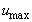fixed. In the following sections, first the steady flow for Reynolds numbers lower than the critical value is presented. An extensive study is done on a wide range of Reynolds numbers with small increments in order to find the critical Reynolds number. This study shows that the critical value of Re is varied from one geometry to another. It has been seen that the critical Reynolds number, Recr is increased with the increase of aspect ratio. This is in agreement with our initial perception that the critical Reynolds number would be lower for blunt bodies (with low aspect ratios) than for the streamlined bodies. It is known that, when the drag is dominated by viscous drag, we say the body is streamlined, and when it is dominated by pressure drag, we say the body is bluff. Therefore, flow around streamlined bodies will experience separation and unsteady boundary layer at higher Reynolds numbers. Critical Reynolds numbers for different geometries based on their aspect ratios are shown in Figure 4. As the Reynolds number increases from this critical value, vortex shedding will occur and flow will enter into an unsteady phase.

Figure 4. Critical Reynolds number vs. Aspect Ratio (AR) of the obstacle (for different geometries from left to right: H2, H1, ST, L1 and L2)

Discussion on the flow patterns for the unsteady regime is presented in section 4.2 and the flow characteristics are discussed in depth. Finally, the vortex shedding phenomena and a study of the Strouhal number are presented in section 4.2.1.

4.1. Steady Flow: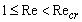The streamlines for the low Reynolds number flow around the diamond-shaped obstacle with different geometries are shown in Figure 5 through Figure 9. The highly viscous flow at low Reynolds number, Re ≤ 1, is known as the creeping flow. This steady flow will persist without separation while the viscous forces are largely dominant. Here, flow around different geometries is shown for the creeping flow at Re = 1 and for a Reynolds number of about half of the critical value for that particular section.

As the Reynolds number increases, viscous forces will decrease until the laminar boundary layers will experience separation. In this region, a steady recirculation region will appear with two vortices that are placed symmetrically on each side of the wake behind the obstacle. Due to the sharp corners of this diamond-shaped obstacle, points of separation are fixed at these edges on top and bottom of the obstacle.

Reynolds number for the steady solution of vortices behind the obstacle is set at a value about half of the critical limit. As a result, the streamlines are shown at different Reynolds numbers based on different critical values, Recr for five geometries.

4.2. Unsteady Flow:With the increase of Reynolds number from the critical limit, set of vortices elongate and become unstable. Therefore, at the end of this wake, transverse oscillations will appear and this will cause the generation of waves along the trail. Source of this instability has been studied for the case of circular cylinder and it was said to be originated in the Hopf bifurcation phenomenon as stated by Williamson . For the case of rectangular cylinder, less argument is available in the literature while a critical value of about Re ≈ 60 ± 5 has been determined by Klekar and Patankar  and also in Breuer et al. . It has been found that this limit depends on flow parameters such as blockage ratio and therefore in the present study, different critical values have been found for various geometries that were examined. The well-known phenomenon of Von Kármán vortex street or in general vortex shedding will appear with further increase of Re when the formation of eddies will occur. Wavelength of vortex shedding will decrease as the Reynolds number rises. In Figure 10 through 14, the streamlines for the flow around diamond-shaped obstacle are presented at the critical Reynolds number for the particular geometry and at Re = 100, 200 for all different cases, except for L2 case at Re = 150, 200.

Final Reynolds number at which the flow has been simulated is set to be Re = 200. The reason for this limit can be sought in the change of principal behaviors in the flow pattern with further increase of the Reynolds number from this particular limit. The frequency of vortex shedding will rise and therefore the laminar shedding will no longer remain 2-dimensional. Further studies in 3-dimensional case has proved this statement that the flow at Reynolds numbers above Re = 200 will experience 3-dimensional instabilities and vortices. Also, in 2-D simulations, deviations from fully periodic structures have been recorded which will restate the above theory. It should also be noted that Reynolds number based on the height of the inlet boundary has already reached the value of Re = 1000 and therefore further increase of Reynolds number would need special treatment for the turbulence flow parameters.

In the case of fully oscillating flow in 2-D simulations, vorticity contours will give a better representation of the Von Kármán vortex street. Therefore, these contour lines are presented in Figure 15 for all different geometrical cases at Re = 200.

4.2.1. Vortex Shedding and Strouhal Number

In the case of unsteady flow around the diamond-shaped obstacle, an important quantity has to be taken into account and that is the Strouhal number (St) which is computed based on the hydraulic diameter of the cylinder, the frequency of the vortex sheddingand the inlet velocity(10)

In order to measure the frequency of vortex shedding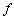, a spectral analysis based on fast Fourier transformation, FFT, has been performed on the time series of oscillating lift forces and the characteristic value of f has been calculated. It was seen that the Strouhal number will increase with rising Re in the range of Recr ≤ Re ≤ 200 which is in good agreement with the literature. This rise in Strouhal number will have a maximum in a particular value which varies from one geometry to another based on different aspect ratios and Recr they have and will decrease again for higher Re until the limit of 2-D simulation, i.e. Re = 200.

Strouhal numbers for unsteady flows in the range of Recr ≤ Re ≤ 200 for different geometries are plotted in Figure 16. The behavior of this non-dimensional number based on the previous statements is in good agreement with the results of previous investigations. Although there is a lack of similar 2-D laminar studies in the case of flow around diamond-shaped obstacles, but it can be noticed that there would be a rise of Strouhal number with an increase in the blockage ratio as presented in the literature, [1, 5]. Also, a local maximum in the St has been reported by various works in the case of flow around rectangular cylinders. The same behavior was recorded for the present case of flow around diamond-shaped obstacles but at different Re based on different blockage ratios for each case.

Figure 16. Computed Strouhal numbers vs. Reynolds number for different obstacles (ST, H1, H2, L1 and L2)

### 5. Conclusions

In the present study, the laminar 2-D flow around a diamond-shaped obstacle was investigated using a finite difference Navier-Stokes solver. Due to the lack of accurate and detailed data in the literature for the present case, the flow was simulated in a range of Reynolds numbers and with five different geometries creating a range of aspect ratios and blockage coefficients. A diamond-shaped obstacle was placed in a confined channel with sufficient distance from both upstream and downstream boundaries. A finite difference Navier-Stokes solver, 2D_NAVISTO, using staggered grid arrangement and Chorin’s projection method was developed as an in-house computer code in order to simulate fluid flow over the obstacles in the confined channel. A mesh dependency test was carried out in order to reach a minimum grid resolution for the required accuracy of the solution. Based on the lack of detailed and similar data for the case of diamond-shaped obstacle, the numerical solver was validated using the results of flow over the rectangular cylinder which has almost similar characteristics based on its sharp corners.

The flow simulation was divided into two different groups. First the steady flow over the obstacles was investigated with the Reynolds numbers range from 1 ≤ Re Recr. The fully viscous or the creeping flow at Re = 1 shows no sign of separation while the flow at higher Reynolds number and below the critical limit, presents two symmetrically placed vortices in the wake of the obstacle. Next, the unsteady flow is simulated with the range of Recr ≤ Re ≤ 200. At the critical Reynolds number Recr, the vortices will become instable and at higher Re, the well-known Von Kármán vortex street will occur. The limit Re = 200 is suggested in the literature for the maximum value at which a 2-D simulation is accurate. Preliminary 3-D studies have shown that at higher Re the vortices will have oscillations in 3-dimension which makes it impossible to achieve accurate results in 2-D simulations.

Diamond-shaped obstacles are altered in order to obtain different aspect and blockage ratios which will have significant effect on the critical Reynolds number Recr. Numerical results prove that bluff bodies will have a lower critical value of Reynolds number than the streamlined bodies. Another study is presented based on the Strouhal number for each case at different Reynolds numbers. It has been found that there is an increase in St with the rise of Re until a maximum value that is found to be different for each geometry.

### References

  Breuer M, Bernsdorf J, Zeiser T, Durst F. Accurate computations of the laminar flow past a square cylinder based on two different methods: lattice-Boltzmann and finite-volume. Int. J. Heat Fluid Flow, 21: 186-196, 2000.In article  Franke, R., Numerische Berechnung der instationnaren Wirbelablosung hinter zylindrischen Korpern. Ph.D. Thesis. University of Karlsruhe, 1991.In article  Okajima A. Strouhal numbers of rectangular cylinders. J Fluid Mech; 123: 379-98, 1982.In article  Klekar, K.M., Patankar, S.V. Numerical prediction of vortex shedding behind square cylinders. Int. J. Numer. Meth. Fluids., 14: 327-341, 1992.In article  Davis RW, Moore EF, Purtell LP. A numerical-experimental study of confined flow around rectangular cylinders. Phys. Fluids, 1984; 27(1): 46-59, 1984.In article  Mukhopadhyay A, Biswas G, Sundararajan T. Numerical investigation of confined wakes behind a square cylinder in a channel. Int. J. Numer. Meth. Fluids, 14: 1473-1484, 1992.In article  Suzuki H, Inoue Y, Nishimura T, Fukutani F, Suzuki K. Unsteady flow in a channel obstructed by a square cylinder rod (crisscross motion of vortex). Int. J. Heat Fluid Flow, 14(1): 2-9,1993.In article  Sohankar A, Norberg C, Davidson L. A numerical study of unsteady twodimensional flow around rectangular cylinders at incidence. Internal Report No. 96/25, Department of Thermo and Fluid Dynamics, Calmers University of Technology, Gothenburg, Sweden; 1996.In article  Nakamura Y, Ohya Y, Ozono S, Nakayama R. Experimental and numerical analysis of vortex shedding from elongated rectangular cylinders at low Reynolds numbers 200-103. J Wind Eng. Ind. Aerodyn. 65: 301-8, 1996.In article  Dutta SH., Panigrahi P.K. Muralidhar K, Experimental Investigation of Flow Past a Square Cylinder at an Angle of Incidence, J Engineering Mechanics, 134(9): 788-803, 2008.In article  Berrone S., Garbero V., Marro M. Numerical simulation of low-Reynolds number flows past rectangular cylinders based on adaptive finite element and finite volume methods. J. Computers & Fluids, 40: 92-112, 2011.In article  Bruno L, Fransos D, Coste N, Bosco A. 3D flow around a rectangular cylinder: a computational study. In: BBAA VI international colloquium on bluff bodies aerodynamics & applications, Milano, Italy; 20-24, 2008.In article  John V, Rang J. Adaptive time step control for the incompressible Navier- Stokes equations. Comput Methods Appl Mech Eng., 199: 514-24, 2010.In article  Xin liang Tian, Muk Chen Ong , Jianmin Yang , Dag Myrhaug. Unsteady RANS simulations of flow around rectangular cylinders with different aspect ratios. J Ocean Engineering 2012; 58 208-216.In article  Kogaki T., Kobayashi T., Taniguchi N., Large eddy simulation of flow around a rectangular cylinder. Fluid Dynamics Research 20(1-6): 11-24, 1997.In article  Regulski W., Szumbarski J., Numerical simulation of confined flows past obstacles -the comparative study of Lattice Boltzmannand Spectral Element Methods, J Arch. Mech., 64: 423-456, 2012.In article  Peric, M., Kessler, R. and Scheuerer, G. Comparison of finite-volume numerical methods with staggered and collocated grids, Computers & Fluids, 16(4): 389-403, 1988.In article  Chorin, A. J., Numerical Solution of the Navier-Stokes Equations, Math. Comp. 22: 745-762, 1968.In article  Williamson, C.H.K., Vortex dynamics in the cylinder wake. Annu. Rev. Fluid Mech. 28: 477-539, 1996.In article